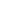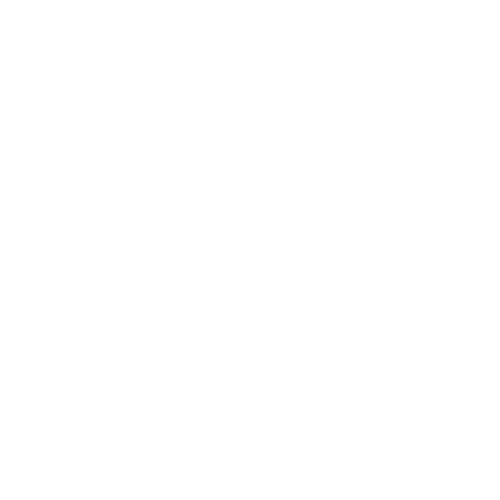JavaScript 101andrewgbliss
9 months agoIn this article we will discuss how React uses JavaScript. What are variables, functions, objects.

. . .

Getting Started

JavaScript has variables, objects, and functions. Let's define what these are and how to use them.

A variable is a place in computer memory that stores a value. For instance if you want to show a user name, That name would be stored in a variable.

An object is a collection of variables. For instance the user object would store the user name, picture, the date when they signed up for the website.

A function is code that will perform a calculation. For instance in our React app, the App.js is a function that returns HTML.

How to write a function

Let's begin by talking about the anatomy of a function. First we want to declare a function by the keyword function, then followed by the name. All functions are declared with parenthesis that enable us to pass in different variables to perform calculations. Last of all the function body is declared by handle bar brackets.

Consider the following function.

return num1 + num2;
}

This function is called addNumbers, it has two variables that we pass in as arguments called num1 and num2. The function body returns the calculation of num1 plus num2.

So if we invoke, or call, this function it will return a calculation.

addNumbers(1, 2) // This would return 3
addNumbers(10, 20) // This would return 30

How to use variables

Now let's go over how to use variables. Variables are essential to programming languages because they store information about who is using the app, and calculations to show the user certain information.

Let's declare a variable called name.

let name = 'Johnny';

We start off by declaring a variable with the keyword let. After the keyword let we give it the name of the variable. Then we assign the variable with the equal operator to the string of Johnny.

We can also creates variables that store numbers.

let amount = 100;
let rate = 10;
let total = amount * rate;

This creates an amount and rate variable and then creates a total variable by multiplying amount and rate together.

How to use objects

Objects are collections that store variables. We use them to group like data together.

let user = {
id: 1,
name: 'Johnny',
age: 25,
picture: 'https://www.gravatar.com/johnny'
};

We can create this object to store all sorts of variables all related to the user that logged in to the website.

To access these variables we use a dot separator.

user.name = 'Johnny';
user.age = 35;

This object in JavaScript is referred to as JSON (JavaScript Object Notation).

What are anonymous functions?

Anonymous functions are just like normal function except they do not have a name. So if we create a click handler for a button in HTML like this:

<button
onClick={() => {
}}

The onClick handler will call this anonymous arrow function anytime the button is clicked.

() => {
// This is the anatomy of an anonymous function.
// do something here
}

Recap

We went over functions, variables, and objects. With these building blocks we can create useful applications that store user data and perform calculations.

Next Lesson

We will talk about how to use functions to create React components.andrewgbliss
9 months ago